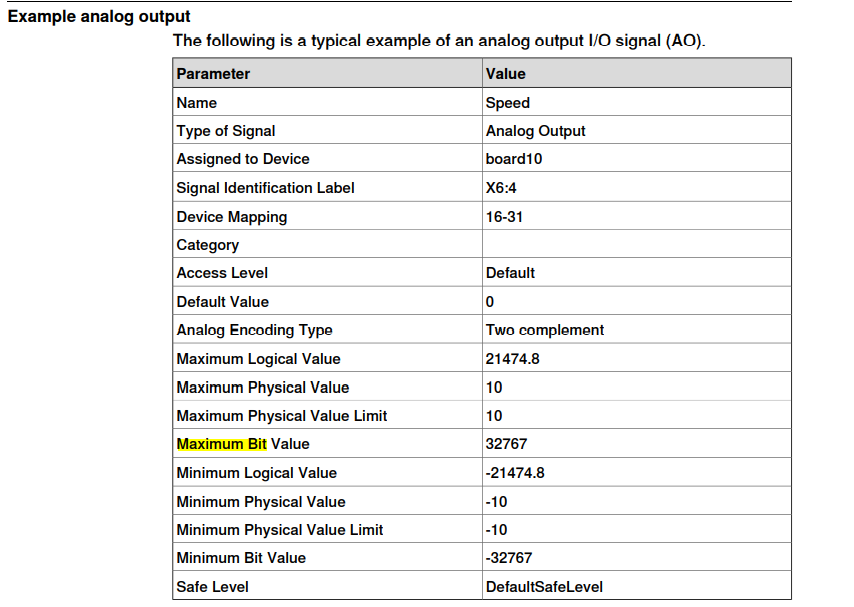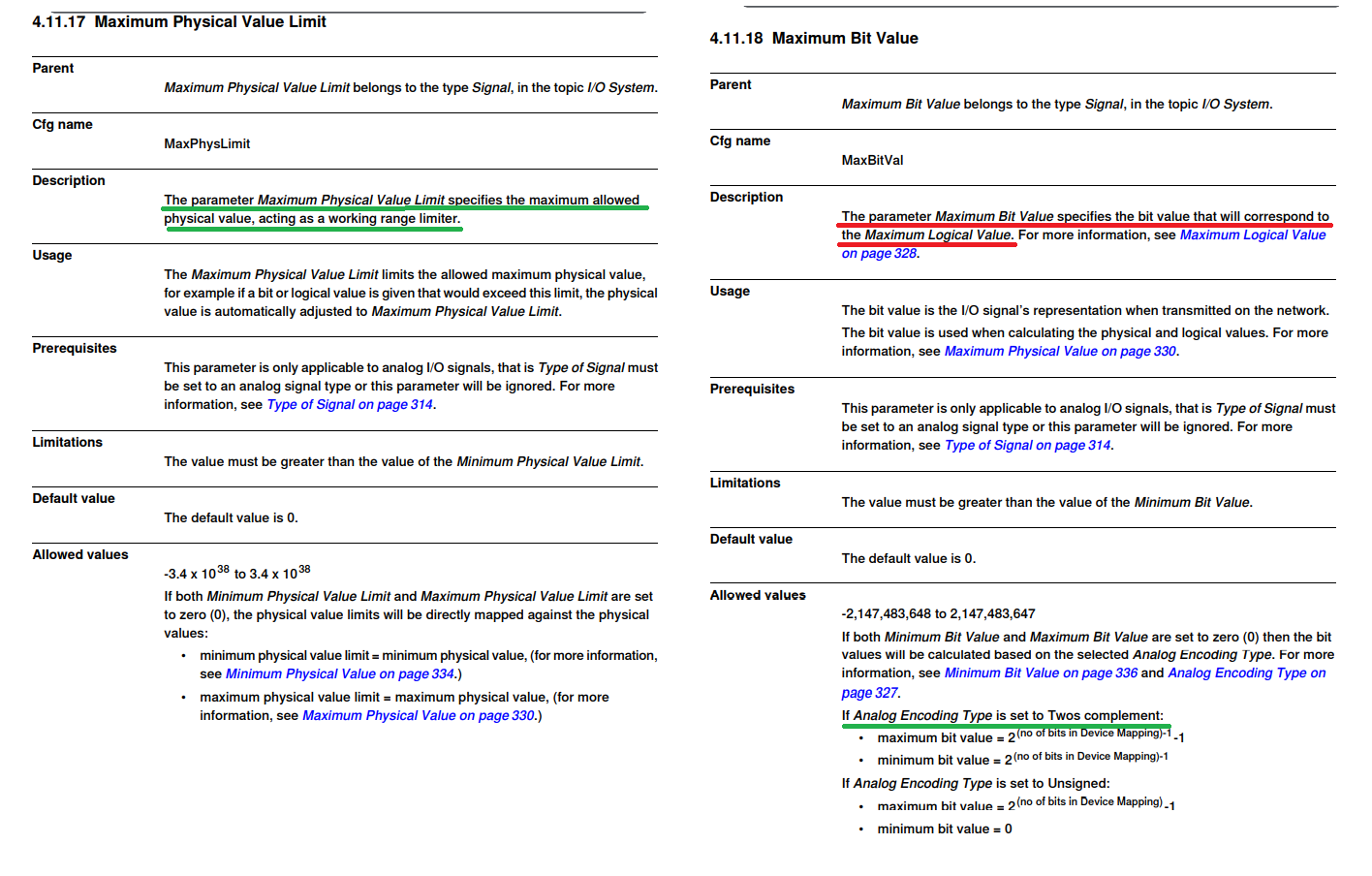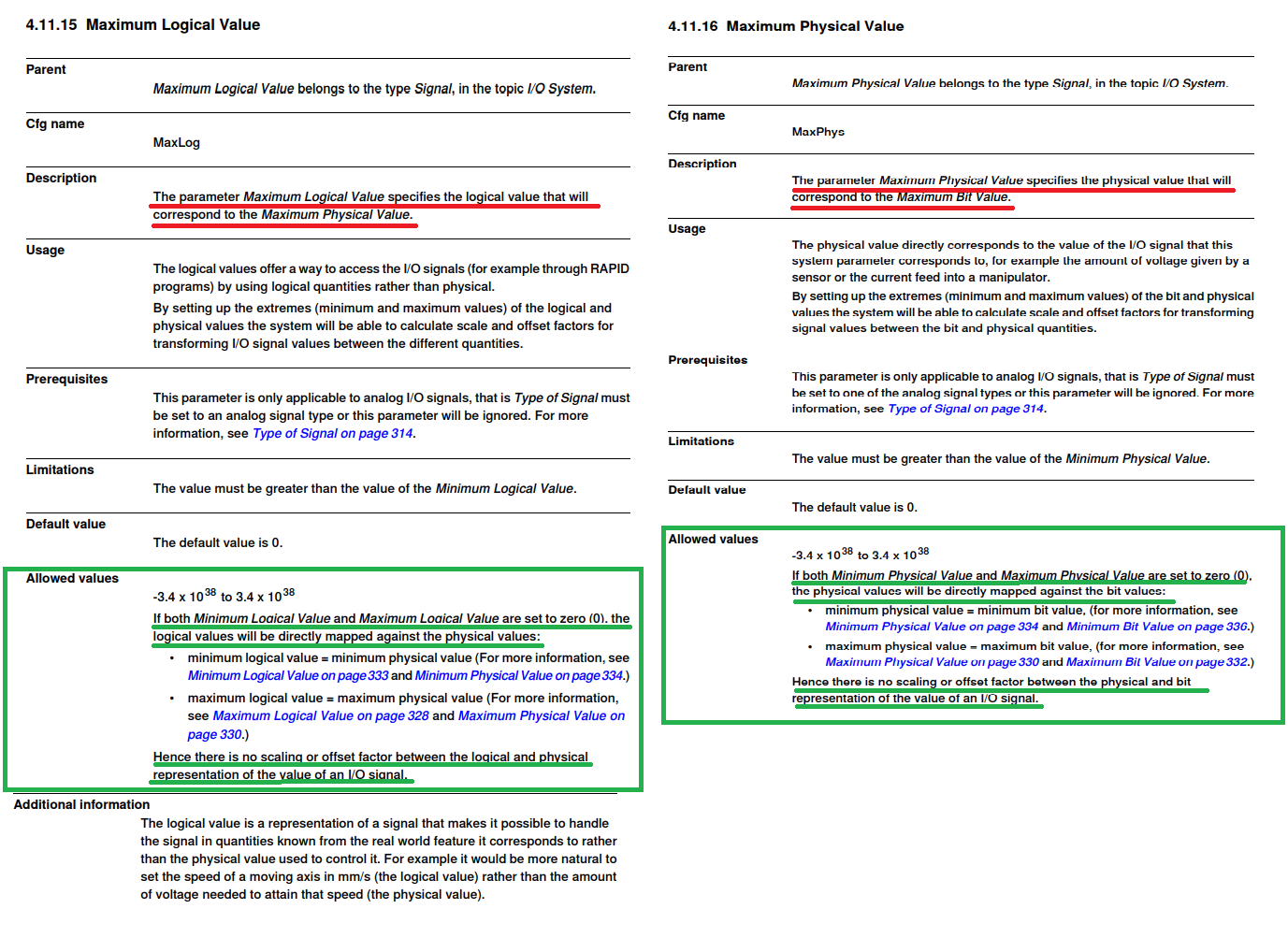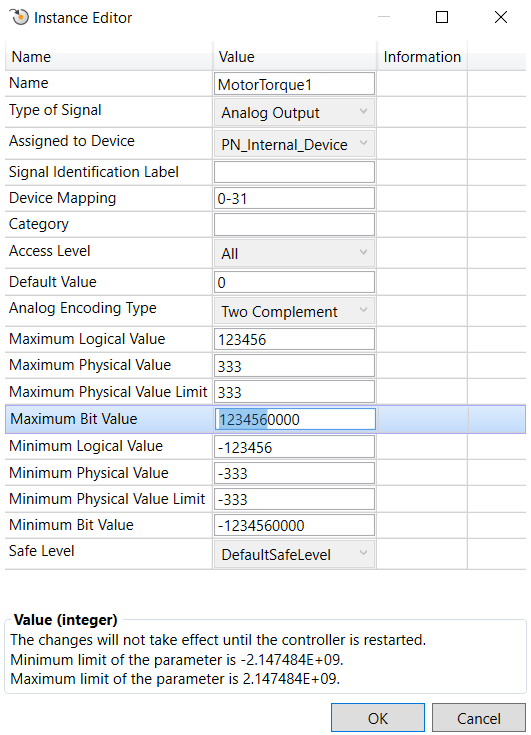Analog I/O signal mapping for PROFINET

Hello everyone,

We need to extract MotorTorques (but in general num RAPID types values) from the PROFINET IRC5 device to my PCL

We managed to established communication between PROFINET device and controller but we bumped into some difficulties in understanding the device mapping for a proper conversion, this is our example at the moment:The doc I followed is contained into:  Technical reference manual - System parameters.

The core info should be the following in terms of our given example:We should have understood the Maximum (Minimum) Bit Value: we would like 32 bit values for our num value mapping, we set them to +/- ((2^31)-1) (if Two complement encoding type is selected).

Concerning the others, Max (min) logical, physical, physical limit have been set 0 according to the doc we underlined in the green box, but here we're not sure (also by considering the example proposed in the documentation where different values were given).

We're also a bit confused about the Analog encoding type meaning (Two complement vs Unsigned): it is clear the semantic use of this according to the physical quantity to be represented, however the Max (min) logical, physical, physical limit: are +/- (3.4 * 10^38), which are the limits of 32 bit IEEE754 floating point convention (Single-precision floating-point format - Wikipedia), whereas AFAIK Two complement is in the domain of integers numbers.

Note: I found this related issue Analog output signal — ABB Robotics User Forums even though I was not able to grasp entirely the solution found, anyway let me know if I need to take actions to avoid duplication problems.

I thank you in advance for any insight on this.

• Hi all,

by looking better at the documentation I was able to grasp some more details.

The most important mapping is between Logical value and Bit value, in particular by considering symmetric interval (min logical = -max logical, min bit = -max bit):
• max logical value is related to the bounds to be set to our quantity (e.g. in Nm for motor torque)
• max bit value is the maximum integer (2-complement's according to the Analog Encoding type we selected) corresponding to the logical value. Here there was the info I was missing:  in order to transfer a floating point value over the (Profinet in our case) network it is first converted to integer by scaling it according to the precision we would like to attain and then it will be then reconverted to floating point from the other side (PROFINET controller).
An example:Here we can reach up to 123456 Nm motor torque (more than sufficient), then in order to get a precision of 10^-4 (even this more than enough) I have to map the maximum torque to its maximum integer representation: for instance a dummy value of 0.9876543 [Nm] will be scaled to 9876.543 and then truncated to 9876 which will be transferred through the network and then reconverted to the approximation 0.9876 [Nm] (tested on our setup).

Another example to represent the remainder of the problem about the max physical value:Here we wanted to exploit at maximum the max bit value (2^31), so we picked as logical value 2^31/10^4 to get the desired precision and everything seems ok.

As you may notice we changed also the physical value bounds, because as far as we tried it seems it does not impact the conversion (possibly because the logical value is mapped into the physical value without loss, being both of them floating point unlike the max bit value?). In essence, we would like to understand to respect of which we need to set it and when it is useful.

If you have any suggestion about this answer just let me know or if there are flaws in our reasoning that we did not spot.

Thanks again.